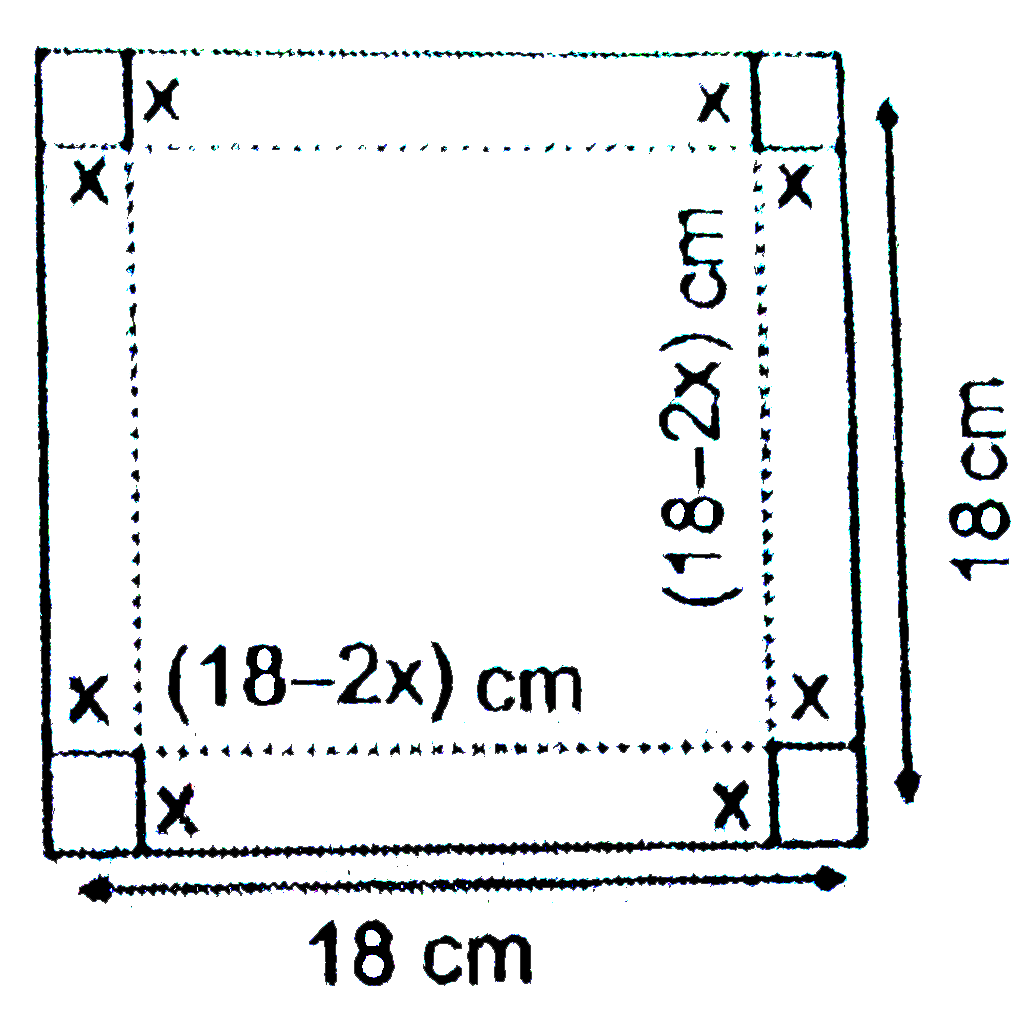# A square piece of tin of side 18 cm is to be made into a box without top, by cutting a square from each corner and folding up the flaps to form the bo

17 views

closed
A square piece of tin of side 18 cm is to be made into a box without top, by cutting a square from each corner and folding up the flaps to form the box. What should be the side of the square to be cut off so that the volume of the box is the maxi

by (94.4k points)
selected

Let the side of square be x and  (0 lt x lt 9) , then the length of box is (18 - 2x), breadth is (18-2x) and height is x . Let V be the volume of the open box formed by folding the flaps of tin.:. V = x ( 18 - 2x)(18-2x)
 = 4x(9-x)^(2)=4x(81+x^(2)-18x)
 = 4(x^(3)-18x^(2)+81x)
 rArr(dV)/(dx) = 4(3x^(2)-35x+81)= 12 (x^(2)-12x+27)
and  (d^(2)V)/(dx^(2)) = 12 (2x- 12) = 24 (x - 6)
For maximum value
 (dV)/(dx) = 0 rArr 12 (x^(2)- 12x + 27) = 0
 rArr x ^(2) - 12x + 27 = 0
 rArr ( x - 3 ) (x - 9) = 0
 rArr x = 3, 9
but x = 9 is impossible so x = 3.
at  x= 3 , ((s^(2)V)/(dx^(2)))_(x = 3 ) = 24 (3 - 6)=- 72 lt 0
 :. x = 3
 rArr  Side of square cut = 3 cm